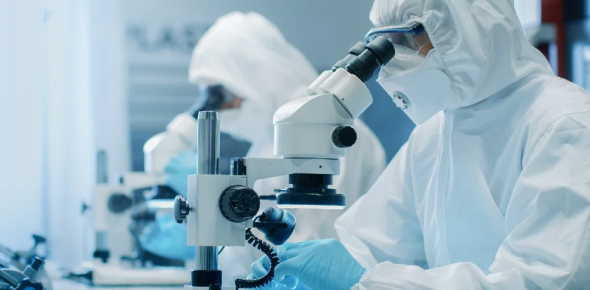# Physics Technical Test Questions And Answers

10 QuestionsSettingsCheck out our online quiz to find a high-order physics technical questionnaire to test your knowledge, prepare for an upcoming exam, or simply enhance your learning.

• 1.
Calculate the nominal, tolerance and clearance in Figure 1: A) Arithmetic  /  B)Statitistical  1.5RSS
• 2.
Given the outside radius Ro, the inside radius Ri the axial force F and a measured friction coefficient µ, derive an expression for the torque T that can be transmitted with this clutch (see figure 2).
• 3.
Does the hole have an effect on the stress? If yes, explain where is the maximum stress? (see figure 3).
• 4.
Two steel bars with identical geometry are subject to an equal load. The first bar has a yield strength of σys = 1000 MPa while the second bar has σys = 500 MPa. The load causes a max. stress of σ = 400 MPa. Which bar deflects more?  (see figure 4).
• 5.
It is known that the machining production of a Impeller hub diameter has a normal distribution. The average of the diameter is ø45.8 and the Sigma is 0.2. Considering that the print spec is ø46+/-0.4.
• 6.
Calculate the equivalent spring rate  of the following spring configurations (A & B).  Consider K1 = 2 N/mm and K2 = 2 N/mm (see figure 6 A & B).
• 7.
Name at least 5 different GD&T
• 8.
Calculate the torque on point (A) when F = 210N and length of rod is 2m and create free body diagram of the stress distribution on the x-section at point A.
• 9.
What case has the highest and the lowest contact stress? Case1: Cylinder-Plane / Case2: Cylinder-Cylinder / Case3: Cylinder-Cylinder
• 10.
In the beam below, with circular x-section, Identify the location point of the highest stress and the possible location of the failure. Create a free body diagram of combined stress of the section.
Related TopicsBack to top